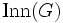# Kirillov orbit method for finite inner-Lazard Lie group

Jump to: navigation, search

This is a slight variation on the Kirillov orbit method for finite Lazard Lie group that works for finite inner-Lazard Lie groups. The range of applicability of this method is slightly larger. For instance, it includes all finite$p$-groups of nilpotency class exactly$p$, most of which are not Lazard Lie groups.

It is capable of providing the degrees of irreducible representations. However, it does not provide as much canonical information about irreducible representations.

## Constructing the action analogous to the coadjoint representation

We start with a group$G$ that is a finite inner-Lazard Lie group. Note that iff$G$ is already a Lazard Lie group, it would be preferable use the usual Kirillov orbit method for finite Lazard Lie group. So the discussion here is useful mostly for the situations where$G$ barely misses being Lazard.

By using the Lazard correspondence up to isoclinism, we can find a Lie ring$L$ that serves as a Lie ring "up to isoclinism" for$G$. This Lie ring is not unique, but it is uniquely determined up to isoclinism of Lie rings.

We now have to define an action of$G$ on$L$ by Lie ring automorphisms. We'll do this by composing the quotient map$G \to \operatorname{Inn}(G)$ with an action of the inner automorphism group$\operatorname{Inn}(G) \cong G/Z(G)$ on$L$, constructed as follows. There is a Lazard correspondence with$\operatorname{Inn}(G)$ the Lazard Lie group and$\operatorname{Inn}(L) \cong L/Z(L)$ the Lazard Lie ring. For any$u \in \operatorname{Inn}(G)$, the corresponding element$\log u \in \operatorname{Inn}(L)$ defines an inner derivation of$L$. Take the formal exponential of this, and use the fact that exponential of derivation is automorphism under suitable nilpotency assumptions to argue that this is an automorphism of$L$. This is the automorphism of$L$ associated with$u \in \operatorname{Inn}(G)$. Basic facts of the Lazard correspondence now show that this defines an action of$\operatorname{Inn}(G)$ on$L$ by automorphisms.

## Identification of coadjoint orbits with irreducible representations

Having constructed an action of$G$ on$L$ by Lie ring automorphisms, we proceed the same way: we use the action to construct a group action by abelian group automorphisms on the Pontryagin dual$\hat{L}$ of$L$ as an additive group. The square roots of the orbit sizes for this action correspond to the irreducible representations.

However, there no longer seems to be a canonical bijection between the irreducible representations and these orbits.